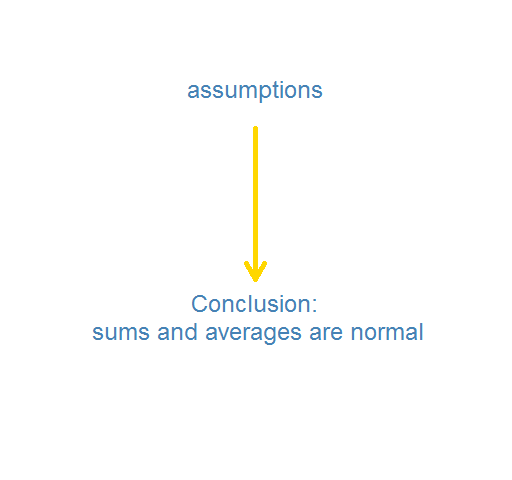# Posts Tagged ‘ Central Limit Theorem ’

## The distribution of financial returns made simple

January 23, 2012
ByWhy returns have a stable distribution As “A tale of two returns” points out, the log return of a long period of time is the sum of the log returns of the shorter periods within the long period. The log return over a year is the sum of the daily log returns in the year.  … Continue reading →

## Example 8.34: lack of robustness of t test with small n

April 12, 2011
ByTim Hesterberg has effectively argued for a larger role for resampling based inference in introductory statistics courses (and statistical practice more generally). While the Central Limit Theorem is a glorious result, and the Student t-test remarkabl...

## Central Limit Theorem A nice illustration of the Central Limit…

October 20, 2010
ByCentral Limit Theorem A nice illustration of the Central Limit Theorem by convolution.in R: Heaviside 0,1,0) }HH

## CLT Standard Normal Generator

April 2, 2010
By$CLT Standard Normal Generator$

I’ve found this standard normal random number generator in a number of places, one of which being from one of Paul Wilmott’s books. The idea is that we can use the Central Limit Theorem (CLT) to easily generate values distributed according to a standard normal distribution by using the sum of 12 uniform random